Courses

# Chemistry Test 6 - Redox Reaction, Nitrogen, Oxygen Family, Carboxylic Acid, Amines Containing Nitrogen Compounds

## 30 Questions MCQ Test JEE Main Mock Test Series 2020 & Previous Year Papers | Chemistry Test 6 - Redox Reaction, Nitrogen, Oxygen Family, Carboxylic Acid, Amines Containing Nitrogen Compounds

Description
This mock test of Chemistry Test 6 - Redox Reaction, Nitrogen, Oxygen Family, Carboxylic Acid, Amines Containing Nitrogen Compounds for JEE helps you for every JEE entrance exam. This contains 30 Multiple Choice Questions for JEE Chemistry Test 6 - Redox Reaction, Nitrogen, Oxygen Family, Carboxylic Acid, Amines Containing Nitrogen Compounds (mcq) to study with solutions a complete question bank. The solved questions answers in this Chemistry Test 6 - Redox Reaction, Nitrogen, Oxygen Family, Carboxylic Acid, Amines Containing Nitrogen Compounds quiz give you a good mix of easy questions and tough questions. JEE students definitely take this Chemistry Test 6 - Redox Reaction, Nitrogen, Oxygen Family, Carboxylic Acid, Amines Containing Nitrogen Compounds exercise for a better result in the exam. You can find other Chemistry Test 6 - Redox Reaction, Nitrogen, Oxygen Family, Carboxylic Acid, Amines Containing Nitrogen Compounds extra questions, long questions & short questions for JEE on EduRev as well by searching above.
QUESTION: 1

### System which suggested Roman numbers to name compounds is called as

Solution: Stock nomenclature for inorganic compounds is a widely used system of chemical nomenclature developed by the German chemist Alfred Stock and first published in 1919.
QUESTION: 2

Solution:
QUESTION: 3

### Suppose a compound contains atoms A,B and C. Theoxidation number of A is 2, B is 5 and C is –2.The possible formula of the compound would be

Solution: When we calculate oxidation number sum should come as zero In c option 2*3+5*2+8*-2=0
QUESTION: 4
Among the following, the strongest oxidising agent is
Solution:
QUESTION: 5
For the redox reaction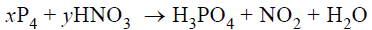Solution:
QUESTION: 6
The oxidation number of sulphur in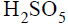is
Solution:
QUESTION: 7
The equivalent weight of Nitrogen in the reaction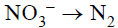is
Solution:
QUESTION: 8
Which of the following ions will undergo
disproportionation reaction ?
Solution:
QUESTION: 9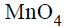is good oxidizing agent in different media,where it changes to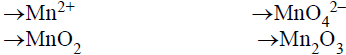The oxidatino number of Mn correspondingly reduces by
Solution:
QUESTION: 10
In the complex Ion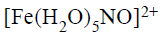Solution:
QUESTION: 11
The IUPAC name of the complex compound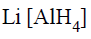is
Solution: The ionization sphere which is lithium Then inside the complex entity u will start with the ligand, therefore, tetrahydride(Because there are 4 hydrogens) and then comes the metal aluminate oxidation number of aluminum is 3. Therefore the name of the compound will be lithium tetrahydride aluminate(III)
QUESTION: 12
The IUPAC name for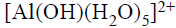is
Solution:
QUESTION: 13
The hyridization states of the central atom in the complex
ions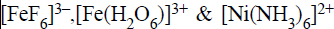are
Solution:
QUESTION: 14
Which of the following statements is correct ?
Solution:
QUESTION: 15
Consider the following spatial arrangements of the
octahedral complex ion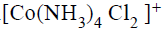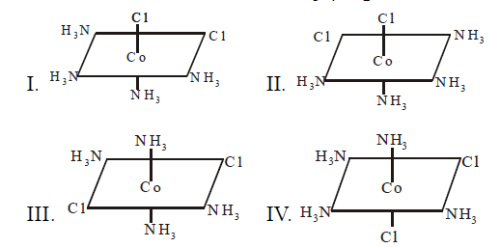Which of the following statement is incorrect
regarding these stuructures ?
Solution:
QUESTION: 16
The chloride ion is detected by the formation of a white
precipitate of AgCl on addition of aqueous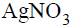solution and by the dissociation of the precipitate on
addition of excess ammonia solution. AgCl dissolves in
aqueous ammonia due to the formation of water woluble
Solution:
QUESTION: 17
One mole of the complex compound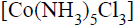gives 3 moles of ions on dissolution in water. One mole of the same complex reacts with two moles ofsolution to yield two moles of AgCl(s).The structure of the complex is
Solution:
QUESTION: 18
Which of the following have the general formula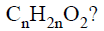Solution:
QUESTION: 19
Which of the following compounds on heating at about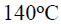gives acetic acid ?
Solution:
QUESTION: 20
In which of the following reactions does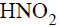act as oxidizing agent ?
Solution:
QUESTION: 21
Consider the following sequence of reactions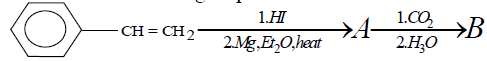The end product (B) is
Solution:
QUESTION: 22
Which one among the following is the strongest reducing
agent ?
Solution:
QUESTION: 23
The general formula of alkanamides is
Solution:
QUESTION: 24
The corect order of decreasing reactivities of the
following compounds towards hydrolysis under
identical conditin is
Solution:
QUESTION: 25
Which of the following compounds exists as a
nonresolvable racemic mixture ?
Solution:
QUESTION: 26
The total number of secondary amines (excluding
stereoisomers) having the molecular formula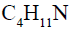is
Solution:
QUESTION: 27
The reaction Of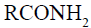with a mixture of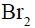and aqueous KOH gives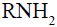as the main product. The
intermediate(s) involved in this reaction is (are)
Solution:
QUESTION: 28

Among the compounds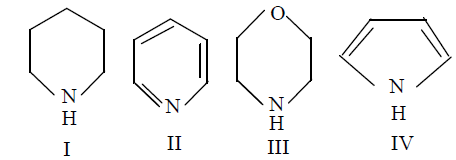the order of basicity is

Solution:
QUESTION: 29
The element that has the least tendency to show
the inert-pair effect is
Solution:
QUESTION: 30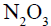is
Solution: